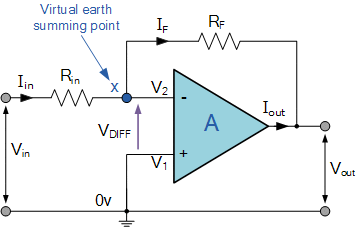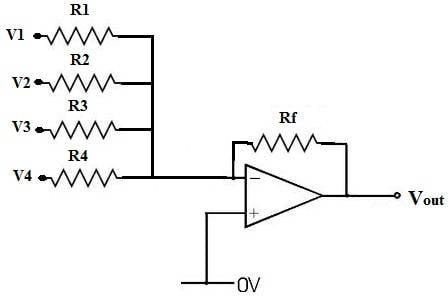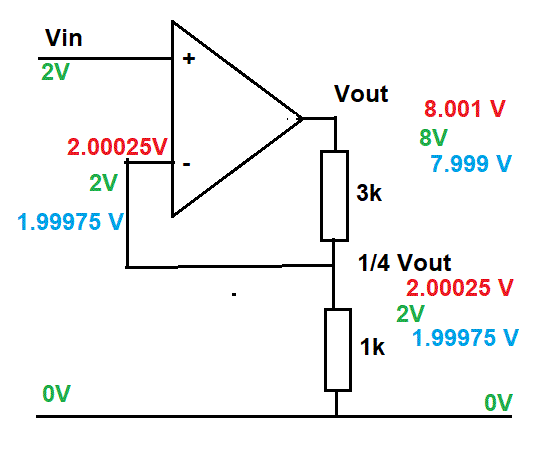# How does an opamp work as non-inverting amplifier

## Homework Statement

I am doing a little bit of research about op amps. What i know so far from the book it that op amp have a very large open loop gain. To reduce the gain to desired amount we need resistor which connect output to invering terminal(negative feedback).

## Homework Equations

How does the op amp work in closed loop? How resistors help to control the magnitude of amplification( assuming the case of non invering amplifier)?i would like to get little bit of intuition about this and possibly avoid the math. Thanks in advance

berkeman
Mentor

## Homework Statement

I am doing a little bit of research about op amps. What i know so far from the book it that op amp have a very large open loop gain. To reduce the gain to desired amount we need resistor which connect output to invering terminal(negative feedback).

## Homework Equations

How does the op amp work in closed loop? How resistors help to control the magnitude of amplification( assuming the case of non invering amplifier)?i would like to get little bit of intuition about this and possibly avoid the math. Thanks in advance
Thread moved to the EE forum...

Typical inverting opamp circuits like this, right?

https://www.electronics-tutorials.ws/opamp/opamp6.gif#### Attachments

anorlunda
Staff Emeritus
We almost always use inverting op amps, so I'll answer that.

The net input to the OP amp is the difference between the incoming signal and the feedback signal (both signals connected via a resistor). The secret is that high amplification forces that difference to be almost zero.

Think of the OP amp gain as a big but finite number G. Then if the output signal is 1 volt, then the net input to the OP amp must be 1/G, which is nearly zero if G is large.

In the diagram below, the net input to the op amp is defined by the connection points marked + and -.#### Attachments

•rishi kesh
Thread moved to the EE forum...

Typical inverting opamp circuits like this, right?

https://www.electronics-tutorials.ws/opamp/opamp6.gif
View attachment 219975
Yes,this is where i have been reading from

CWatters
Homework Helper
Gold Member
The key is that opamps amplify the difference between the + and - inputs. They also have a very large gain. Without feedback that difference would just depend on the input signal and the output would be very large, in fact way too large. Negative feedback reduces the difference at the inputs and as a result it reduces the output to the required level.

The key is that opamps amplify the difference between the + and - inputs. They also have a very large gain. Without feedback that difference would just depend on the input signal and the output would be very large, in fact way too large. Negative feedback reduces the difference at the inputs and as a result it reduces the output to the required level.
So when we have a negative feedback there is a very little difference between the voltages at inverting and non inverting inputs.and since op amps in practical have some finite gain the output will be amplified without rising up extremely high. Am i right?

Merlin3189
Homework Helper
Gold Member
...How does the op amp work in closed loop? How resistors help to control the magnitude of amplification( assuming the case of non invering amplifier)?i would like to get little bit of intuition about this ...
I won't avoid numbers, but perhaps i can keep the maths simple.

First a reminder of how the op=amp uses its two inputs.
If the + input has a higher voltage than the - input, then the output voltage goes up towards +
If the - input has a higher voltage than the + input, then the output goes down.towards - (or zero if there's no -ve voltage rail.)

Here's a non-inverting circuit using an op-amp. It gives a gain of 4 (closed loop gain.)
The output has two resistors between it and 0V, so that the output voltage is split, 1/4 across the 1k resistor and 3/4 across the 3k resistor.
The green writing shows the 2V input and the expected x4 = 8V output.
It shows the 1/4 of output = 2V above the 1k resistor (the other 6V are across the 3k resistor.)
And this 2V is fed back to the inverting input, making it the same as the other input.In red I show the output voltage drifting a little above 4x the input. If you follow the red numbers, you see that 1/4 of this voltage gets back to the inverting input. So now the inverting input (-) is higher than the non-inverting input (+) and it will make the output go lower, which will bring it back down towards 8V.

In blue I show the output drifting a little below 4x the input. Following the blue numbers, 1/4 of the output gets back to the inverting input. Now the + input is higher than the - input, so the output will go up, back towards 8V

When the 2V is first applied to the input, no matter what the output is it will be driven up or down as needed towards 8V. There it will be stable as long as there is no differece (actually a very tiny difference, maybe about 0.00001 V) between the two inputs.

For this sort of amplifier, the actual "closed loop" gain is set by this pair of resistors. Here we feedback 1/4 of the output and gain is 4. If we feedback 1/10 of the output, gain is 10. Feedback 1/20 th of the output, gain is 20.
The less we feed back to the negative (inverting) input, the greater the gain (the fractions were getting smaller, so the gain increased.)
If we feed back all of the output, the gain is 1, so no gain at all - the output is the same as the input. Sounds silly, but it's very useful. We give the input a voltage, but we don't have to give it any current (well just a tiny bit, like 0.0000001 A or less ) and the output gives exactly the same voltage, but able to supply maybe as much 0.1 A, certainly enough to light a LED, flip a relay or drive a nice big transistor to give even more power.

#### Attachments

•jim hardy and rishi kesh
Thank
BTW, here is a nice summary of the benefits of negative feedback from Hyperphysics: http://hyperphysics.phy-astr.gsu.edu/hbase/Electronic/feedn.html

(and your avatar is very scary!)Oh yeah! But for me its cute :P

I won't avoid numbers, but perhaps i can keep the maths simple.

First a reminder of how the op=amp uses its two inputs.
If the + input has a higher voltage than the - input, then the output voltage goes up towards +
If the - input has a higher voltage than the + input, then the output goes down.towards - (or zero if there's no -ve voltage rail.)

Here's a non-inverting circuit using an op-amp. It gives a gain of 4 (closed loop gain.)
The output has two resistors between it and 0V, so that the output voltage is split, 1/4 across the 1k resistor and 3/4 across the 3k resistor.
The green writing shows the 2V input and the expected x4 = 8V output.
It shows the 1/4 of output = 2V above the 1k resistor (the other 6V are across the 3k resistor.)
And this 2V is fed back to the inverting input, making it the same as the other input.
View attachment 219980
In red I show the output voltage drifting a little above 4x the input. If you follow the red numbers, you see that 1/4 of this voltage gets back to the inverting input. So now the inverting input (-) is higher than the non-inverting input (+) and it will make the output go lower, which will bring it back down towards 8V.

In blue I show the output drifting a little below 4x the input. Following the blue numbers, 1/4 of the output gets back to the inverting input. Now the + input is higher than the - input, so the output will go up, back towards 8V

When the 2V is first applied to the input, no matter what the output is it will be driven up or down as needed towards 8V. There it will be stable as long as there is no differece (actually a very tiny difference, maybe about 0.00001 V) between the two inputs.

For this sort of amplifier, the actual "closed loop" gain is set by this pair of resistors. Here we feedback 1/4 of the output and gain is 4. If we feedback 1/10 of the output, gain is 10. Feedback 1/20 th of the output, gain is 20.
The less we feed back to the negative (inverting) input, the greater the gain (the fractions were getting smaller, so the gain increased.)
If we feed back all of the output, the gain is 1, so no gain at all - the output is the same as the input. Sounds silly, but it's very useful. We give the input a voltage, but we don't have to give it any current (well just a tiny bit, like 0.0000001 A or less ) and the output gives exactly the same voltage, but able to supply maybe as much 0.1 A, certainly enough to light a LED, flip a relay or drive a nice big transistor to give even more power.
You explained it very clear now i got it!

CWatters
Homework Helper
Gold Member
So when we have a negative feedback there is a very little difference between the voltages at inverting and non inverting inputs.and since op amps in practical have some finite gain the output will be amplified without rising up extremely high. Am i right?

Yes.

Opamps have such a huge gain that without feedback (open loop) even small input signals would cause the output to "go to the rail". For example if the opamp gain was 10,000 and the input signal was 10mV then the output would try to be 10mV * 10,000 = 1000V. That's not usually possible because the supply rails to the opamp are typically only +/- 15V.

If feedback is used to limit the gain of the overall circuit to say 100 then the same 10mV input signal would produce an output of 1V which is well within the +/-15V supply rails.

In this case the difference between +ve and -ve inputs on the opamp would be about 1V/10,000 = 10uV. That's so small it can usually be treated as 0V. and is sometimes called a "virtual earth".

•jim hardy and rishi kesh
jim hardy
Gold Member
Dearly Missed
So when we have a negative feedback there is a very little difference between the voltages at inverting and non inverting inputs.and since op amps in practical have some finite gain the output will be amplified without rising up extremely high. Am i right?

Two excellent answers precede this post. I think you have it.

This memory aid works for me:
"It is the duty of the circuit designer to surround the operational amplifier with a circuit that lets it make its inputs equal."

An "operational amplifier" is just a high gain amplifier.
When we surround it with a circuit that lets it make its inputs equal we have built an 'operational amplifier circuit'
whose input and output are related by some mathematical function. It performs that math operation on its input. The math function is defined by the input and feedback parts of the circuit.

If an op-amp circuit doesn't look easy to figure out, here's what i do:
Write KVL equations for voltages at amplifier's + and - inputs as functions of Vout, Vin, and components in the feedback circuit. Note these might be differential equations if there's capacitive or inductive reactance involved.
Set those equations equal to one another and solve for Vout/Vin.

If the op-amp[ can't make its inputs equal, the circuit has ceased to "operate" ..

old jim

Last edited:
•rishi kesh